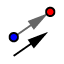# Translating by a Vector

## DIRECTIONS:

In the applet below, 1) Construct a triangle. 2) Use the VECTOR TOOLto construct any vector whose terminal points DOES NOT lie on top of its initial point. 3) Display the label of the triangle's 3 vertices and vector you've just constructed. Use the "Name and Value" option when showing the label. 4) Use the TRANSLATE BY VECTOR TOOLto translate the triangle by the given vector.

## 5)

The coordinates for points are (x, y) and the notation for a vector is <a, b>. How can we use this information to write a rule to get the coordinates for the image (also called triangle A'B'C').

## 6)

Use the rule above to answer the question. A triangle has the coordinates A(0,-5), B(2,2), and C(7,0). The vector is <1,5>. What are the coordinates for the image (triangle A'B'C')?

When you're done (or if you're unsure of something), feel free to check by watching the quick silent screencast below the applet.